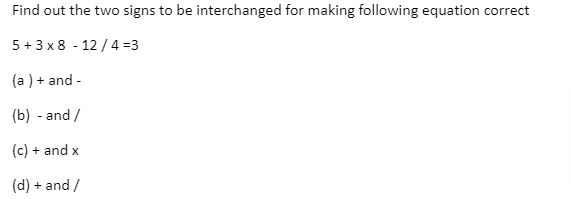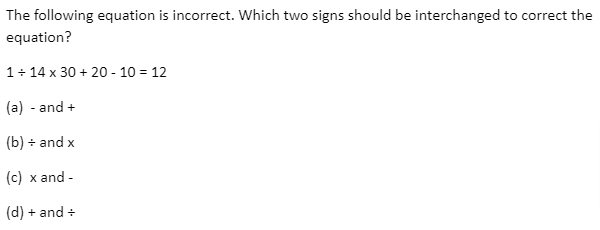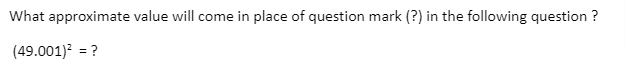## Mathematical Operations Reasoning MCQs for Entrance exams

Mathematical Operations MCQ Quiz- Practice Mathematical Operations reasoning MCQ Question suitable for various Competitive Exams. Here we have compiled Mathematical Operations MCQ Questions with solutions for those students who are preparing for various competitive exams. Practice these Mathematical Operations MCQ questions to increase your confidence and mental ability.

1. Given intercharges :
Signs + and x and numbers 4 and 5.
a.
b.
c.
d.

2. Can you Solve 7 + 7 ÷ 7 + 7 x 7 + 7 − 7 ÷ 7 + 7 x 7 =
a.
b.
c.
d.

3. If "A" denotes "subtracted from", "B" denotes "added to", "C" denotes "divided by", "D" denotes "multiplied by", then which of the following statement is correct?
a.
b.
c.
d.

4. Which of the following interchanges in signs and numbers will make the given equation correct?
8 x 9 + 2 = 74
a.
b.
c.
d.

5. The last two digits of 2151415?
a.
b.
c.
d.

6. If + means +, – means x, + means + and x means then 36 x 12 + 4 + 6 + 2 – 3 = ?
a.
b.
c.
d.

7. Which one of the four interchanges in signs and numbers would make the given equation correct?
3 + 5 – 2 = 4
a.
b.
c.
d.

8. If x stands for ‘addition’, + stands for ‘subtraction’, + stands for ‘multiplication’ and – stands for ‘division’, then 20 x 8 + 8 – 4 + 2 – ?
a.
b.
c.
d.

9. It being given that : > denotes +, < denotes denotes =,>
a.
b.
c.
d.

10. If × means ÷, - means ×, ÷ means + and + means- than (3 - 15 ÷ 19) × 8 + 6 = ?
a.
b.
c.
d.

11. If ‘+’ means ‘divided by’, ‘–‘ means ‘added to’, ‘×’ means ‘subtracted from’ and ‘÷’ means ‘multiplied by’, then what is the value of 15 ÷ 12 – 36 + 3 ?
a.
b.
c.
d.

12. If P denotes ‘multiplied’ by’, T denotes ‘subtracted’ from’, M denotes ‘add to’ and B denotes ‘divided by’, then 28 B 7 P 8 T 6 M 4 =?
a.
b.
c.
d.

13.a.
b.
c.
d.

14. The solution of 3(2u + v) = 7 uv and 3(u + 3v) = 11 uv is _____
a.
b.
c.
d.

15. In a certain code language, '-' represents'+', '+' represents 'x', 'x' represents '÷' and '÷'represents '-'. Find out the answer to the following question.
2 - 12 + 10 x 5 ÷ 12 = ?
a.
b.
c.
d.

16. If 25 + 5 ÷ 2 = 40 , and 35 + 5 ÷ 2 = 60 , then 45 + 5 ÷ 2 = ?
a.
b.
c.
d.

17. In a certain code language, '+' represents 'x', '-' represents '+', 'x' represents '÷' and '÷' represents '-'. What is the answer to the following question?
25 + 10 ÷ 240 x 16 = ?
a.
b.
c.
d.

18.a.
b.
c.
d.

19. If "÷" denotes "multiplied by", "+" denotes "subtracted from", "x" denotes "added to" and "–" denotes "divided by",then
12 – 6 + 28 x 3 ÷ 9 = ?
a.
b.
c.
d.

20. If ‘+’ stands for ‘×’, ‘–‘ for ‘÷’, ‘×’ for ‘–‘ and ‘÷’ for ‘+’, find the value of 12 + 90 – 5 × 9 ÷ 3.
a.
b.
c.
d.

21. The value of -4-(-10) is how much greater then the value of -10-(-4) ?
a.
b.
c.
d.

22.a.
b.
c.
d.

23. If Q means 'add to', J means 'multiply by', T means 'substract from' and K means 'divide by' then 30 K 2 Q 3 J 6 T 5 = ?
a.
b.
c.
d.

24. If the signs + and / and the numbers 2 and 4 are interchanged, which one of the following four equations would be correct?
a.
b.
c.
d.

25. If – means x, x means +, + means 4, and + means then 40x 12 + 3- 6 + 60 = ?
a.
b.
c.
d.